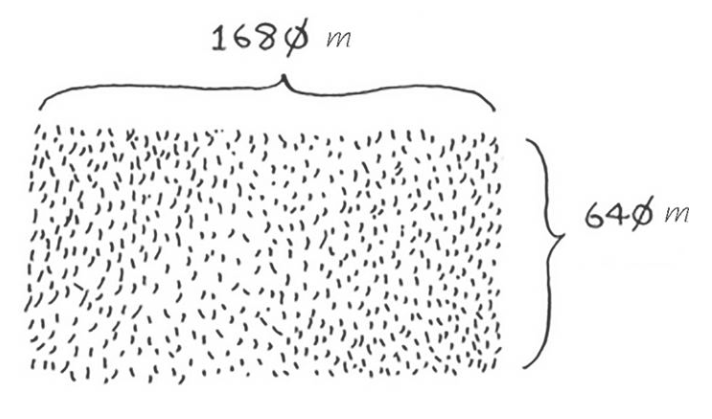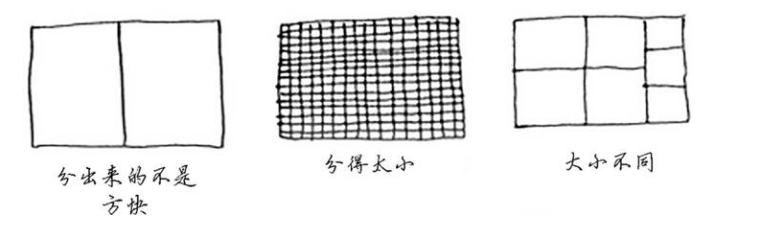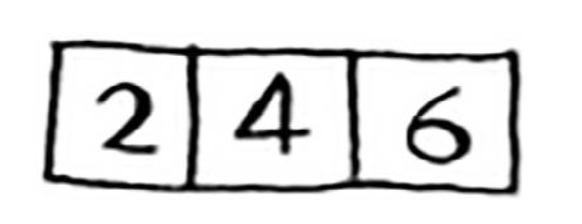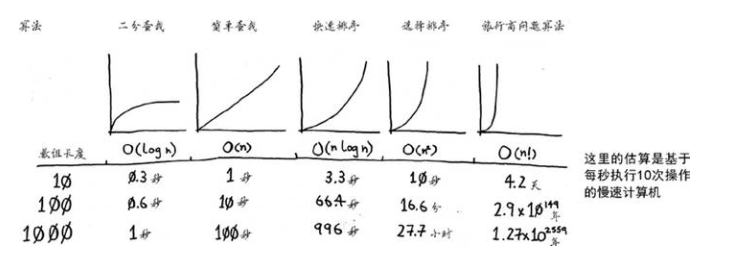# 算法图解之快速排序## 分而治之(又称D&C)(1)D&C算法是递归的;
(2)使用D&C解决问题的过程包括两个步骤:
a.找出基线条件，这种条件必须尽可能简单;
b.不断将问题分解(或者说缩小规模)，直到符合基线条件;

`package cn.pratice.simple;public class Euclid {  public static void main(String[] args) {  int m = 63;  int n = 18;  int remainer = 0;  while(n!=0) {remainer = m % n;m = n;n = remainer;  } System.out.println(m); }}``package cn.pratice.simple;public class Euclid {  public static void main(String[] args) {  int []num = new int[] {2,4,6};  int total = 0;  for (int i = 0; i < num.length; i  ) {total  = num[i]; }  System.out.println(total); }}`

## 快速排序

`package cn.pratice.simple;public class QuickSort {  //声明静态的 getMiddle() 方法，该方法需要返回一个 int 类型的参数值，在该方法中传入 3 个参数 public static int getMiddle(int[] list,int low,int high) { int tmp = list[low];//数组的第一个值作为中轴(分界点或关键数据) while(low<high) {while(low<high && list[high]>tmp) { high--;}list[low] = list[high];//比中轴小的记录移到低端while(low<high&&list[low]<tmp) { low  ;}list[high]=list[low];//比中轴大的记录移到高端  } list[low] = tmp;//中轴记录到尾 return low; }  //创建静态的 unckSort() 方法，在该方法中判断 low 参数是否小于 high 参数，如果是则调用 getMiddle() 方法，将数组一分为二，并且调用自身的方法进行递归排序 public static void unckSort(int[] list,int low,int high) { if(low<high) {int middle = getMiddle(list,low,high);//将list数组一分为二unckSort(list,low,middle-1);//对低字表进行递归排序unckSort(list,middle 1,high);//对高字表进行递归排序  } }  //声明静态的 quick() 方法，在该方法中判断传入的数组是否为空，如果不为空，则调用 unckSort() 方法进行排序 public static void quick(int[] str) {  if(str.length>0) {//查看数组是否为空unckSort(str,0,str.length-1);  } }  //测试 public static void main(String[] args) { int[] number = {13,15,24,99,14,11,1,2,3};  System.out.println("排序前:");  for (int i : number) {System.out.print(i " ");  } quick(number); System.out.println("\r排序后:");  for (int i : number) {System.out.print(i " ");  } }}`

## 再谈大O表示法`package cn.pratice.simple;import java.util.Arrays;public class MergeSort { private static void mergeSort(int[] original) {  if (original == null) {throw new NullPointerException("The array can not be null !!!");  }  int length = original.length;  if (length > 1) {int middle = length / 2;int partitionA[] = Arrays.copyOfRange(original, 0, middle);// 拆分问题规模int partitionB[] = Arrays.copyOfRange(original, middle, length);// 递归调用mergeSort(partitionA);mergeSort(partitionB);sort(partitionA, partitionB, original);  } } private static void sort(int[] partitionA, int[] partitionB, int[] original) {  int i = 0;  int j = 0;  int k = 0;  while (i < partitionA.length && j < partitionB.length) {if (partitionA[i] <= partitionB[j]) { original[k] = partitionA[i]; i  ;} else { `